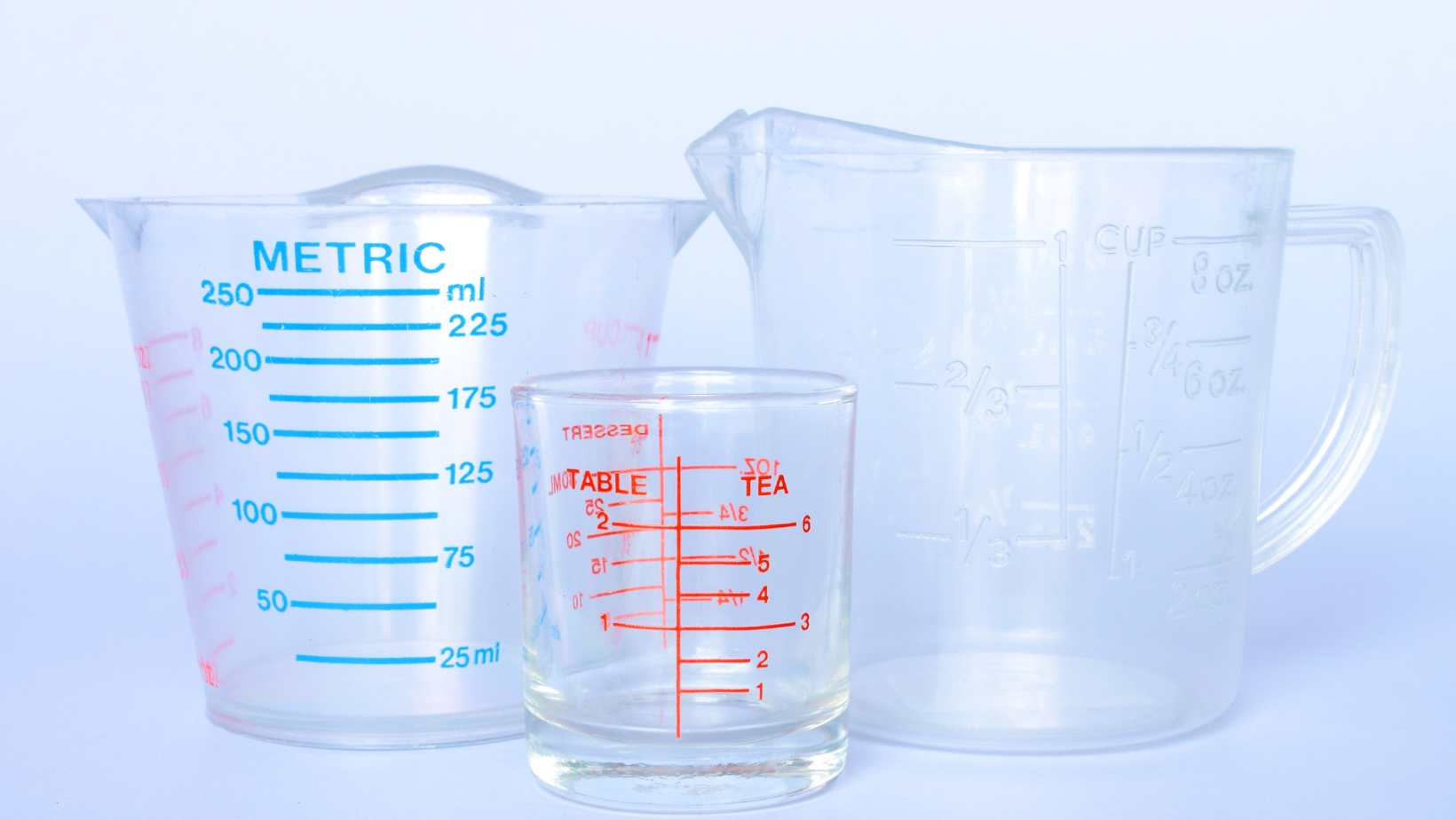# Find Out The Conversion: How Many ML Are in a LBIf you’ve ever found yourself wondering how many milliliters are in a pound, you’re not alone. Converting between different units of measurement can be confusing, especially when dealing with volume and weight. In order to answer the question of how many ml are in a lb, we need to understand the relationship between milliliters (ml) and pounds (lb).

Firstly, it’s important to note that milliliters are a unit of volume, while pounds are a unit of weight. They measure two different properties. However, if we’re considering an equivalent substance with known density, we can make an approximate conversion.

## How Many ML Are In A LB

When it comes to understanding the conversion between milliliters (ml) and pounds (lb), it’s important to grasp the basic concept. While these are two different units of measurement – one volume and one weight – there is a way to convert ml to lb.

To begin, we need to establish that milliliters measure volume, while pounds measure weight. This means that ml represents the amount of space occupied by a substance, while lb measures the force exerted on an object due to gravity. So, converting ml to lb requires us to take into account the density of the substance being measured.

### The Conversion Equation: How to Calculate ML to LB

To calculate ml to lb, you’ll need two essential pieces of information: the density of the substance in question and its volume in milliliters. The equation for converting ml to lb is:

*Weight (lb) = Volume (ml) × Density*

For example, let’s say we have 500 ml of a liquid with a density of 0.9 g/ml. To convert this into pounds, we can use the equation mentioned earlier:

*Weight (lb) = 500 ml × 0.9 g/ml*

Now, since there are approximately 454 grams in a pound, we need an additional step:

*Weight (lb) = (500 ml × 0.9 g/ml) / 454 g/lb*

By performing this calculation, we can determine how many pounds our initial quantity of liquid equates to.

### Common Conversions: ML to LB Examples

To provide further clarity on how conversions from milliliters (ml) to pounds (lb) work in practical scenarios, here are a few common examples:

1. Converting water:

– Water has a density close enough to 1 g/ml for practical purposes.

– Therefore, 1000 ml of water would roughly equal 2.2 pounds.

2. Converting milk:

– The density of milk varies depending on factors such as fat content.

– For whole milk with a density around 1.03 g/ml, 500 ml would be approximately 1.13 pounds.

3. Converting cooking oil:

– Cooking oils have different densities based on their composition.

– Olive oil, for instance, has a density around 0.92 g/ml, so 250 ml would be about 0.51 pounds.

Remember that the actual conversion may vary slightly depending on the specific substance being measured and its exact density value.

In conclusion, understanding how to convert milliliters to pounds involves considering the density of the substance in question and using a simple equation to calculate the weight in pounds. By applying this knowledge and using examples from common substances like water, milk, and cooking oil, you can easily grasp how many pounds are equivalent to a given amount of milliliters.

## The Relationship Between Volume And Weight

When it comes to understanding the relationship between volume and weight, it’s important to consider the specific units of measurement being used. In this case, we are looking at milliliters (ml) and pounds (lb). So, how many ml are in a lb?

To convert between milliliters and pounds, we need to take into account the density of the substance being measured. Different substances have different densities, which means that their weight can vary for a given volume.

For example, water has a density of approximately 1 gram per milliliter. Since there are 454 grams in a pound, we can conclude that there are roughly 454 ml in a pound of water.

However, this conversion factor will not hold true for other substances with different densities. For instance, oil is less dense than water and therefore weighs less for the same volume. On the other hand, substances like lead or gold have higher densities and weigh more.

To accurately convert between ml and lb for substances other than water, you would need to know the specific density of that substance.

In conclusion:

– The number of milliliters in a pound depends on the density of the substance being measured.

– For water specifically, there are approximately 454 ml in a pound.

– To convert between ml and lb accurately for other substances, you would need to know their specific densities.

Amanda is the proud owner and head cook of her very own restaurant. She loves nothing more than experimenting with new recipes in the kitchen, and her food is always a big hit with customers. Amanda takes great pride in her work, and she always puts her heart into everything she does. She's a hard-working woman who has made it on her own, and she's an inspiration to all who know her.